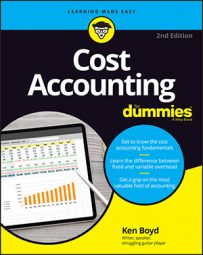##### Cost Accounting For DummiesWhen cost accounting, the more accurately you allocate fixed overhead costs, the more accurately your product’s total costs are reflected. If total cost is accurate, you can add a profit and calculate an accurate sale price. To more accurately allocate fixed overhead you use cost pools and cost allocations to compute a cost allocation rate.

## Compute a cost allocation rate in cost accounting

Say you make car tires. Your cost pool for fixed overhead includes machine depreciation, utility costs, and salary costs for your security guard. The annual budgeted costs total \$120,000, and you have 20,000 total machine hours budgeted. Use these formulas and these numbers to compute your cost allocation rate:

```Budgeted cost allocation rate = \$120,000 cost ÷ 20,000 machine hours
Budgeted cost allocation rate = \$6 per machine hour```

You determine that a budgeted quantity per unit (per tire) is 30 minutes. Here is your budgeted fixed manufacturing overhead cost per unit:

```Fixed overhead cost per unit = .5 hours per tire x \$6 cost allocation rate per machine hour
Fixed overhead cost per unit = \$3```

Each tire has direct costs (steel belts, tread) and \$3 in fixed overhead built into it.

Next, apply actual costs and the static budget. Take the total cost pool of \$120,000 and simply divide it over 12 months. Your monthly static budget is \$120,000 ÷ 12 months. That’s \$10,000 per month.

## Calculate flexible budget variances rate in cost accounting

You can now calculate a fixed overhead flexible-budget variance (sometimes referred to as a spending variance). A flexible budget changes as activity levels (sales, production) change. Because fixed costs do not change within a relevant range, there is no adjustment of budgeted fixed costs from a static to a flexible budget. This holds true as long as actual costs are within the relevant range.

Bad news. The static budget simply took the annual cost pool amount of \$120,000 and divided by 12 months. But say total actual costs for April are \$11,000. You have a variance, and it is not favorable. Here’s the formula:

```Fixed overhead flexible budget variance = actual cost – static budget
Fixed overhead flexible-budget variance = \$11,000 - \$10,000
Fixed overhead flexible-budget variance = \$1,000 unfavorable variance```

The variance is unfavorable because your actual spending (\$11,000) was more than the static budget (\$10,000). If you’re dealing with a spending variance, a positive number is an unfavorable variance. You spent more than planned.

## Use production volume variances rate in cost accounting

An efficiency variance means that you used either more or less of the input (material, labor) than you planned. The variance reflects how efficiently you used your inputs to create a product or service.

For fixed overhead, there isn’t an efficiency variance. The fixed cost is what it is. You incur the same amount of fixed costs regardless of how efficiently you produce your goods. If your actual production is higher or lower than planned, it doesn’t change your flexible budget total for fixed overhead variance.

Whatcha gonna do? Instead of efficiency variance, fixed overhead variance uses something called a production-volume variance. Because fixed costs are fixed, the production volume variance measures how much output you got for the fixed costs you put in. The focus is on the output, not the amount of costs you put in (the input). This variance reveals how efficient you were at producing goods using a fixed level of budgeted costs.

This variance compares budgeted fixed overhead and allocated fixed overhead, based on actual output. (That’s a mouthful.) Here’s another way of saying it: You take the budgeted fixed overhead and apply it to actual output.

For fixed overhead analysis only, budgeted fixed overhead, flexible budget, and static budget all mean the same thing. This is because fixed costs don’t changes as the level of volume changes. That relationship is true when you operate within the relevant range.

Assume actual tire production for April is 3,500. The table shows budgeted fixed overhead applied to actual output.

 Budgeted cost allocation rate (A) \$6 per unit Budgeted machine hours per tire (B) 0.5 (30 minutes) Actual tires produced (C) 5,000 Budgeted overhead applied (A x B x C) \$15,000

Now that you have the budgeted fixed overhead applied, you can compute production volume variance:

```Production volume variance = budgeted fixed overhead - budgeted fixed overhead applied
Production volume variance = \$10,000 - \$15,000
Production volume variance = (\$5,000) favorable variance```

The negative variance is favorable because this is a cost variance. Spending less is what you want to do.

What this variance tells you is that even though you planned to spend only \$10,000 in fixed costs, you were able to produce more tires for the same budgeted amount of money. In theory, producing 5,000 tires should have cost you \$15,000 in budgeted fixed costs. However, you made them within the \$10,000 budget. This saved you \$5,000, because you were more efficient at producing your goods than you planned.

What you saw for fixed overhead analysis were two variances: the flexible budget variance and the production volume variance.